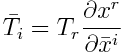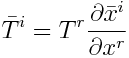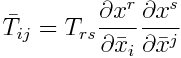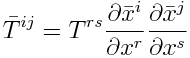# Tensors - Part 4: Behavior Under Change of Coordinates

### The fourth installment of the Tensors series covers the flavors and mixer of tensors.

· Big Data Zone · Opinion
Save
2.49K Views

in the first post in this series, i mentioned several apparently unrelated things that are all called tensors, one of these being objects that behave a certain way under changes of coordinates. that’s what we’ll look at this time.

in the second post , we said that a tensor is a multilinear functional. a k -tensor takes k vectors and returns a number, and it is linear in each argument if you hold the rest constant. we mentioned that this relates to the “box of numbers” idea of a tensor. you can describe how a k -tensor acts by writing out k nested sums. the terms in these sums are called the components of the tensor.

tensors are usually defined in a way that has more structure. they vary from point to point in a space, and they do so in a way that in some sense is independent of the coordinates used to label these points. at each point, you have a tensor in the sense of a multilinear functional, but the emphasis is usually on the changes of coordinates.

## components, indexes, and coordinates

tensors in the sense that we’re talking about here come in two flavors: covariant and contravariant. they also come in mixtures; more on that later.

we consider two coordinate systems, one denoted by x ‘s and another by x ‘s with bars on top. the components of a tensor in the x -bar coordinate system will also have bars on top. for a covariant tensor of order one, the components satisfyfirst of all, coordinates are written with superscripts. so x r is the r coordinate, not x to the power r . also, this uses einstein summation notation: there is an implicit sum over repeated indexes, in this case of r .

the components of a contravariant tensor of order one satisfy similar but different equation:the components of a covariant tensor are written with subscripts and the components of a contravariant tensor with superscripts. in the equation for covariant components, the partial derivatives are with respect to the new coordinates, the x bars. in the equation for contravariant components, the partial derivatives are with respect to the original coordinates, the x ‘s. mnemonic: when the indexes go down (covariant tensors) the new coordinates go down (in the partial derivatives). when the indexes go up, the new coordinates go up.

for covariant tensors of order two, the change of coordinate formula is:here there the summation convention says that there are two implicit sums, one over r and one over s .

the contravariant counterpart says:in general, you could have tensors that are a mixture of covariant and contravariant. a tensor with covariant order p and contravariant order q has p subscripts and q superscripts. the partial derivatives have x -bars on bottom corresponding to the covariant components and x -bars on top corresponding to contravariant components.

## relation to multilinear functionals

we initially said a tensor was a multilinear functional. a tensor of order k takes k vectors and returns a number. now we’d like to refine that definition to take two kinds of vectors. a tensor with covariant order p and contravariant order q takes p contravariant vectors and q covariant vectors. in linear algebra terms, instead of simply taking k elements of a vector space v , we say our tensor takes p vectors from the dual space v * and q vectors from v .

## relation to category theory

you may be familiar with the terms covariant and contravariant from category theory , or its application to object oriented programming . the terms are related. as michael spivak explains, “it’s very easy to remember which kind of vector field is covariant, and which is contravariant — it’s just the opposite of what it logically ought to be [from category theory].”

Topics:
tensorflow, functions, tensors

Published at DZone with permission of John Cook.

Opinions expressed by DZone contributors are their own.